# Which Model Makes Sense?

###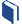Resources for this lesson:

> Glossary> Calculator Resources> Teacher Resources: Instructional NotesCompare your response with the solution below:

## Create and Analyze

1. Upon initial inspection, a linear model appears to be a good fit.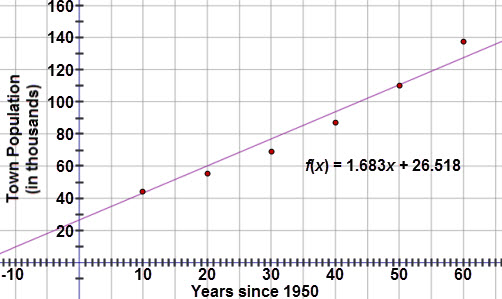## Test and Confirm

1. The correlation coefficient is 0.981 and the scatter plot looks fairly linear.  However, when examining the residual plot, there is a clear pattern to the residuals, meaning that a linear model is not the best fit.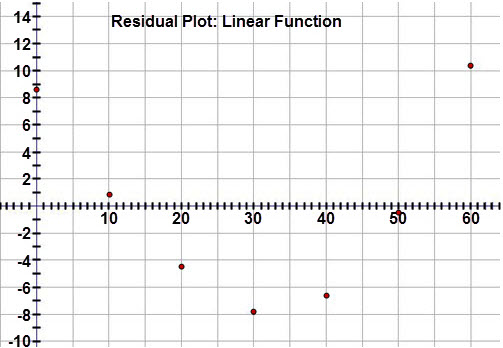1. While the quadratic curve seems to fit the data well, the residual plot reveals a clear pattern. This indicates that a quadratic model is not the best fit.
2.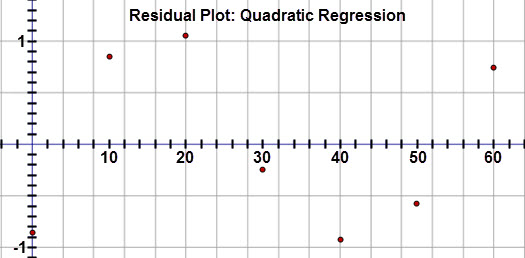3. Since the data is continuing to increase, the only other model that makes sense is an exponential model.  The exponential curve of best fit equation is y = 35.156(1.023)x.

The residuals for the exponential model are:

 Years since 1950 Residual value (in thousands) 0 10 20 30 40 50 60 0.08 0.093 0.106 −0.469 −0.172 0.369 −0.011

The residual graph is random and close to zero, confirming that the exponential model is a good fit for the data.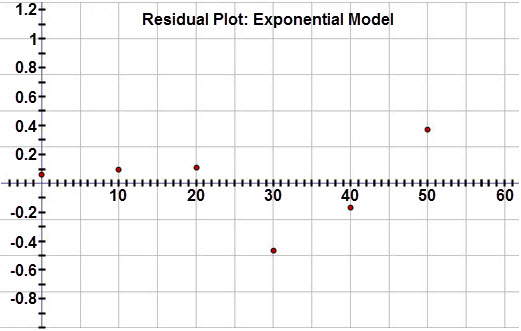It appears that an exponential model is the best fit for this data set.